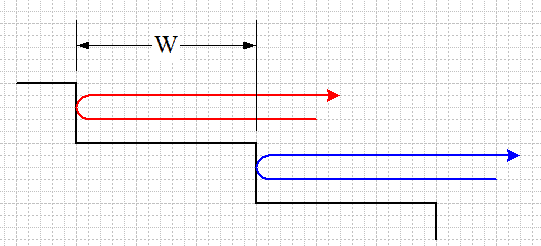# Sound Wave Problem: Frequency of Note in Amphitheater

• i_hate_math
In summary, the conversation discusses how a handclap in an amphitheater produces a periodic series of pulses that create a played note. The question is to find the frequency of the perceived note, given the width of the terraces and the speed of sound in air. The solution involves understanding the movement and speed of the sound waves, the path length difference between returning pulses from adjacent terraces, and using relevant equations to calculate the frequency. The final answer is 177.35 Hz.

## Homework Statement

A handclap on stage in an amphitheater sends out sound waves that scatter from terraces of width w = 0.967 m (see the figure). The sound returns to the stage as a periodic series of pulses, one from each terrace; the parade of pulses sounds like a played note. (a) Assuming that all the rays in the figure below are horizontal, find the frequency at which the pulses return (that is, the frequency of the perceived note). (b) If the width w of the terraces were smaller, would the frequency be higher or lower? (Note: Assume the speed of sound in air = 343 m/s.)

## Homework Equations

S = Sm*cos(kx-vt) maybe?

## The Attempt at a Solution

I am clueless for this question, can someone suggest an approach? I don't know what the theory id behind this
Thanks

#### Attachments

•Untitled.png
16.3 KB · Views: 805
Last edited:
Sound from a single source is bouncing off of a series of surfaces whose distances from the source are separated by a regular fixed increment.

What's moving? How fast does it move? What's the path length difference for sound returning from adjacent terraces? What then is the time difference between returning pulses from adjacent terraces? Knowing the time interval between return pulses, what's the frequency?

Surely you can quote relevant equations that pertain to velocity, distance, and time? How about period and frequency?

You should be able to make an attempt.

•i_hate_math
gneill said:
Sound from a single source is bouncing off of a series of surfaces whose distances from the source are separated by a regular fixed increment.

What's moving? How fast does it move? What's the path length difference for sound returning from adjacent terraces? What then is the time difference between returning pulses from adjacent terraces? Knowing the time interval between return pulses, what's the frequency?

Surely you can quote relevant equations that pertain to velocity, distance, and time? How about period and frequency?

You should be able to make an attempt.
The sound waves are moving at 343m/s, the time difference is T=w/343 = 0.967/343=0.002819...
Hence the frequency is f=1/T=354.705274 Hz
Does that look right?

i_hate_math said:
The sound waves are moving at 343m/s, the time difference is T=w/343 = 0.967/343=0.002819...
Hence the frequency is f=1/T=354.705274 Hz
Does that look right?
Almost. Take a close look at the extra distance traveled by the reflected waves from successive terraces. Remember, the waves are reflected so they travel the same path forward and back:•i_hate_math
gneill said:
Almost. Take a close look at the extra distance traveled by the reflected waves from successive terraces. Remember, the waves are reflected so they travel the same path forward and back:
View attachment 100394
Oh right! Shame on me for not seeing that.
It should be T=2w/343 = 2*0.967/343=0.0056384..
and f=1/T=177.35 Hz

That looks good.

•i_hate_math
gneill said:
That looks good.
Thank you very much for your help!